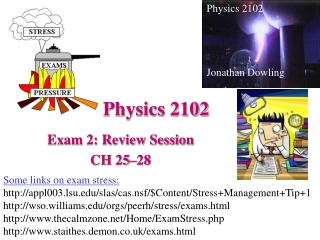DownloadDownload PresentationPhysics 2102

# Physics 2102

Download Presentation## Physics 2102

- - - - - - - - - - - - - - - - - - - - - - - - - - - E N D - - - - - - - - - - - - - - - - - - - - - - - - - - -
##### Presentation Transcript

1. Physics 2102 Jonathan Dowling Physics 2102 Exam 2: Review Session CH 25–28 Some links on exam stress: http://appl003.lsu.edu/slas/cas.nsf/\$Content/Stress+Management+Tip+1 http://wso.williams.edu/orgs/peerh/stress/exams.html http://www.thecalmzone.net/Home/ExamStress.php http://www.staithes.demon.co.uk/exams.html

2. Exam 2 • (Ch 26) Capacitors: capacitance and capacitors; caps in parallel and in series, dielectrics; energy, field and potential in capacitors. • (Ch 27) Current and Resistance: current, current density and drift velocity; resistance and resistivity; Ohm’s law. • (Ch 28) Circuits: emf devices, loop and junction rules; resistances in series and parallel; DC single and multiloop circuits, power; RC circuits.

3. C = ke0A/d Capacitors E = s/e0 = q/Ae0 E = V d q = C V C = e0A/d C=e0ab/(b-a)

4. V = i R E = Jr Current and resistance i = dq/dt R = rL/A Junction rule r = r0(1+a(T-T0))

5. Multiloop Single loop DC Circuits Loop rule V = iR P = iV

6. Resistors and Capacitors ResistorsCapacitors Key formula: V=iR Q=CV In series: same current same charge Req=∑Rj 1/Ceq=∑1/Cj In parallel: same voltage same voltage 1/Req=∑1/Rj Ceq=∑Cj

7. Capacitors and Resistorsin Series and in Parallel • What’s the equivalent resistance (capacitance)? • What’s the current (charge) in each resistor (capacitor)? • What’s the potential across each resistor (capacitor)? • What’s the current (charge) delivered by the battery?

8. Time constant: RC RC Circuits i(t)=dq/dt

9. Capacitors: Checkpoints, Questions

10. Problem 25-21 When switch S is thrown to the left, the plates of capacitor 1 acquire a potential V0. Capacitors 2 and 3 are initially uncharged. The switch is now thrown to the right. What are the final charges q1, q2, and q3 on the capacitors?

11. Current and Resistance: Checkpoints, Questions

12. Problem 26-56 A cylindrical resistor of radius 5.0mm and length 2.0 cm is made of a material that has a resistivity of 3.5x10-5Wm. What are the (a) current density and (b) the potential difference when the energy dissipation rate in the resistor is 1.0W?

13. Circuits: Checkpoints, Questions

14. 1. HRW7 27.P.018.  Figure 27-33 shows five 5.00 resistors. (Hint: For each pair of points, imagine that a battery is connected across the pair.) Fig. 27-33 (a) Find the equivalent resistance between points F and H. (b) Find the equivalent resistance between points F and G.

15. 5. HRW7 27.P.046.  In an RC series circuit, E = 17.0 V, R = 1.50 M, and C = 1.80 µF. (a) Calculate the time constant. (b) Find the maximum charge that will appear on the capacitor during charging. (c) How long does it take for the charge to build up to 10.0 µC?

16. v F L Magnetic Forces and Torques

17. Ch 28: Checkpoints and Questions

18. 5. HRW7 28.P.024.  In the figure below, a charged particle moves into a region of uniform magnetic field , goes through half a circle, and then exits that region. The particle is either a proton or an electron (you must decide which). It spends 160 ns in the region. (a) What is the magnitude of B? (b) If the particle is sent back through the magnetic field (along the same initial path) but with 3.00 times its previous kinetic energy, how much time does it spend in the field during this trip?